# Op Amp Circuit

Operational amplifier or Op Amp as they are generally called are linear DC amplifiers. An op amp is a three-terminal device, one called the inverting terminal, one non-inverting terminal and the other is called the output terminal.

Below, you can see a pin diagram of a typical Op amp.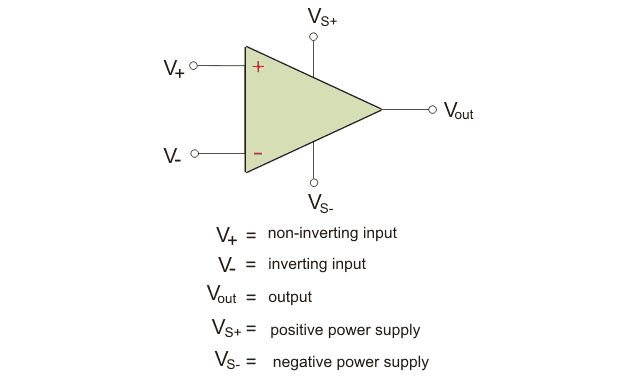An ideal Op amp has the following characteristics:

• An infinite open-loop voltage gain.
• Infinite input impedance.
• Zero output impedance.
• Infinite common-mode rejection ratio.
• Infinite bandwidth.
• Zero offset voltage.

However, real op amps have characteristics a little deviated from what has been stated above. You can read more about the characteristics about op amps in other articles that we have written.
In this article, we are going to learn about the internal circuit of an op-amp IC. Along with that, we are also going to learn about the ac equivalent circuit of op-amp.

## Pin Diagram of an Op Amp IC

The op-amp IC we are going to discuss here is IC 741. It is an 8 pin IC. The pin configuration of IC 741 is given below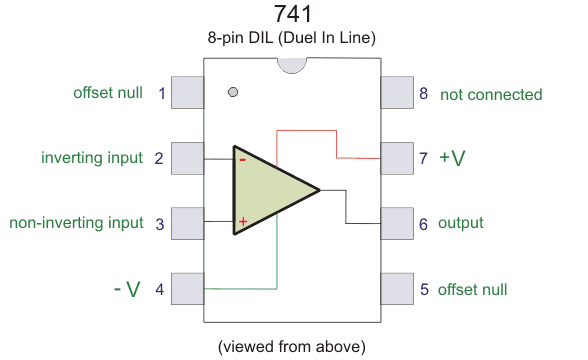PIN 1 – Offset Null
PIN 2 – Inverting input
PIN 3 – non-inverting input
PIN 4 – negative voltage supply
PIN 5 – offset null
PIN 6 – output
PIN 7 – positive voltage supply
PIN 8 – not connected

The internal circuit diagram of an op-amp IC is given below: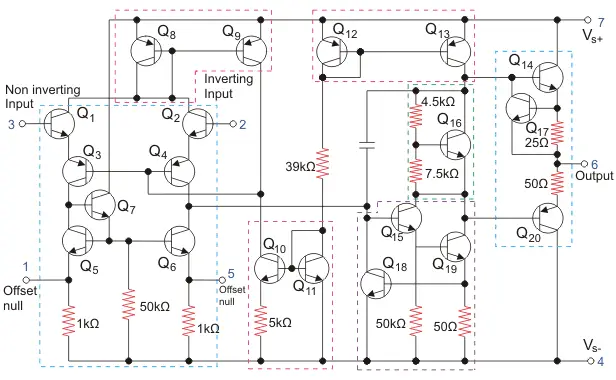As you can see above, the non-inverting terminal and offset null are located at the left. The different terminals are marked in different colors. Op-amp is a collection of transistors and resistances as you can see from the picture.

Op-amp is used for different purposes. The 741 IC can be used as

Here is a circuit diagram of Op-amp IC being used as a comparator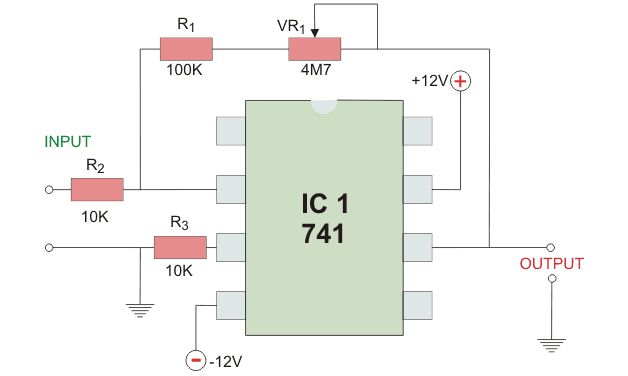Comparator as the name suggests compares two things. In this case, it compares two analogs signals. There will be one voltage signal which will be used as a reference and another signal which is to be compared. Op-amp will then determine which of the two signals has a greater magnitude.

## AC equivalent Circuit of Op amp

The equivalent circuit of an op-amp is the circuit where the op-amp parameters are represented in terms of physical components. Such a representation is very helpful for analysis purposes.
The equivalent circuit of the op amp is given below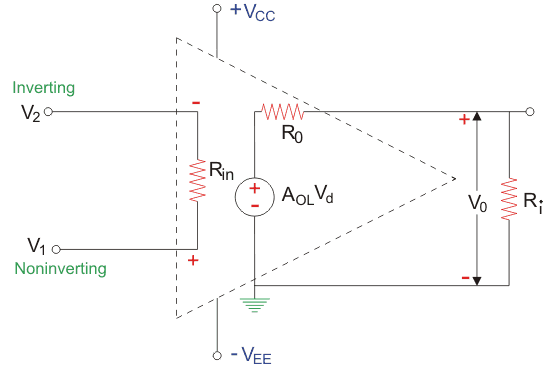Circuit parameters like the internal resistance, output resistance, voltage gain are all represented by circuit components like Rin, Rout, etc.
V1 – non-inverting input voltage with respect to ground;
V2 – inverting input voltage with respect to ground;
Vee – negative supply voltage;
Vcc – positive supply voltage;
Vout – AOLVd = AOL(v1-v2);
Vd – the difference between inverting voltage and non-inverting voltage;
Ri – internal resistance of Op-amp;
R0 – output resistance of op-amp;
AOL – open-loop voltage gain.

The output voltage as you know is directly proportional to the difference between the input voltage.

The voltage AOLVd is the Thevenin’s equivalent voltage source whereas Ro is the Thevenin equivalent resistance as seen from the output terminal. This is the AC equivalent circuit of an Op amp. This is very useful for analyzing various op-amp circuits.

Want To Learn Faster? 🎓
Get electrical articles delivered to your inbox every week.
No credit card required—it’s 100% free.